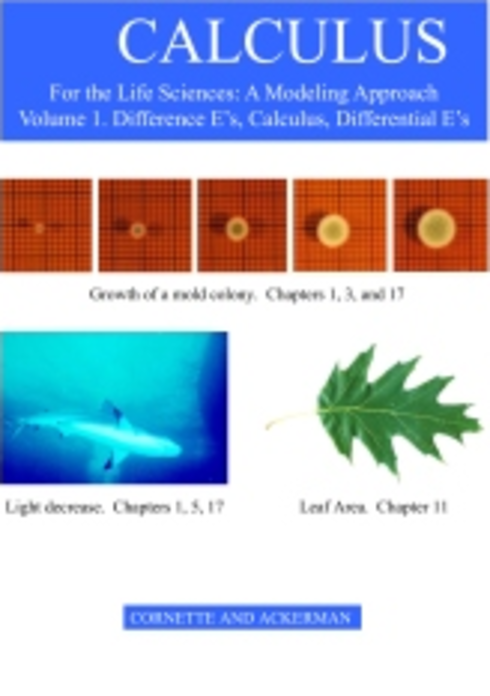# Calculus for the Life Sciences: A Modeling Approach Volume 1

(1 review)James L. Cornette, Iowa State University

Ralph A. Ackerman, Iowa State University

ISBN 13: 9781467976664

Publisher: A.T. Still University

Language: English

## Conditions of UseAttribution-NonCommercial-NoDerivs
CC BY-NC-ND

## Reviews

• 1 Mathematical Models of Biological Processes
• 2 Functions
• 3 The Derivative
• 4 Continuity and the Power Chain Rule
• 5 Derivatives of the Exponential and Logarithm Functions
• 6 Derivatives of Products, Quotients, and Compositions
• 7 Derivatives of Trigonometric Functions
• 8 Applications of Derivatives
• 9 The Mean Value Theorem and Taylor's Polynomials
• 10 Partial Derivatives and the Diffusion Equation
• 11 The Integral
• 12 The Fundamental Theorem of Calculus
• 13 Applications of the Fundamental Theorem of Calculus

## Ancillary Material

• Submit ancillary resource

Our writing is based on three premises. First, life sciences students are motivated by and respond well to actual data related to real life sciences problems. Second, the ultimate goal of calculus in the life sciences primarily involves modeling living systems with difference and differential equations. Understanding the concepts of derivative and integral are crucial, but the ability to compute a large array of derivatives and integrals is of secondary importance. Third, the depth of calculus for life sciences students should be comparable to that of the traditional physics and engineering calculus course; else life sciences students will be short changed and their faculty will advise them to take the 'best' (engineering) course.

In our text, mathematical modeling and difference and differential equations lead, closely follow, and extend the elements of calculus. Chapter one introduces mathematical modeling in which students write descriptions of some observed processes and from these descriptions derive first order linear difference equations whose solutions can be compared with the observed data. In chapters in which the derivatives of algebraic, exponential, or trigonometric functions are defined, biologically motivated differential equations and their solutions are included. The chapter on partial derivatives includes a section on the diffusion partial differential equation. There are two chapters on non-linear difference equations and on systems of two difference equations and two chapters on differential equations and on systems of differential equation.